# Pandas Groupby – Sort within groups

Pandas Groupby is used in situations where we want to split data and set into groups so that we can do various operations on those groups like – Aggregation of data, Transformation through some group computations or Filtration according to specific conditions applied on the groups.

In similar ways, we can perform sorting within these groups.

Example 1: Let’s take an example of a dataframe:

 `df ``=` `pd.DataFrame({``'X'``: [``'B'``, ``'B'``, ``'A'``, ``'A'``], ` `                   ``'Y'``: [``1``, ``2``, ``3``, ``4``]}) ` ` `  `# using groupby function ` `df.groupby(``'X'``).``sum``() `

Output: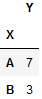Let’s pass the sort parameter as False.

 `# using groupby function  ` `# with sort ` `df.groupby(``'X'``, sort ``=` `False``).``sum``() `

Output: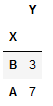Here, we see a dataframe with sorted values within the groups.

Example 2:
Now, let’s take an example of a dataframe with ages of different people. Using sort along with groupby function will arrange the transformed dataframe on the basis of keys passes, for potential speedups.

 `data ``=` `{``'Name'``:[``'Elle'``, ``'Chloe'``, ``'Noah'``, ``'Marco'``,   ` `                 ``'Lee'``, ``'Elle'``, ``'Rachel'``, ``'Noah'``],   ` `        ``'Age'``:[``17``, ``19``, ``18``, ``17``,   ` `               ``22``, ``18``, ``21``, ``20``]}    ` ` `  `df ``=` `pd.DataFrame(data)  ` `df `

Output: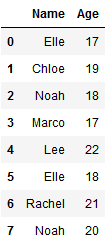Let’s group the above dataframe according to the name

 `# using groupby without sort ` `df.groupby([``'Name'``]).``sum``() `

Output: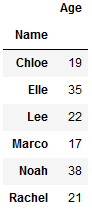Passing the sort parameter as False

 `# using groupby function  ` `# with sort ` `df.groupby([``'Name'``], sort ``=` `False``).``sum``() `

Output: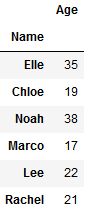Example 3:
Let’s take another example of a dataframe that consists top speeds of various cars and bikes.
We’ll try to get the top speeds sorted within the groups of vehicle type.

 `import` `pandas as pd ` ` `  ` `  `df ``=` `pd.DataFrame([(``'Bike'``, ``'Kawasaki'``, ``186``), ` `                   ``(``'Bike'``, ``'Ducati Panigale'``, ``202``), ` `                   ``(``'Car'``, ``'Bugatti Chiron'``, ``304``),  ` `                   ``(``'Car'``, ``'Jaguar XJ220'``, ``210``), ` `                   ``(``'Bike'``, ``'Lightning LS-218'``, ``218``),  ` `                   ``(``'Car'``, ``'Hennessey Venom GT'``, ``270``), ` `                   ``(``'Bike'``, ``'BMW S1000RR'``, ``188``)],  ` `                  ``columns ``=``(``'Type'``, ``'Name'``, ``'top_speed(mph)'``)) ` ` `  `df `

Output: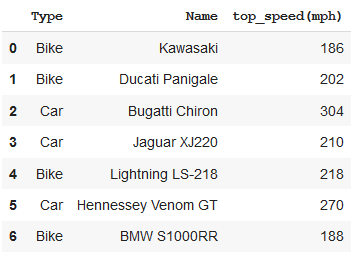After Using the groupby function

 `# Using groupby function ` `grouped ``=` `df.groupby([``'Type'``])[``'top_speed(mph)'``].nlargest() ` ` `  `# using nlargest() function will get the  ` `# largest values of top_speed(mph) within ` `# groups created ` `print``(grouped) `

Output: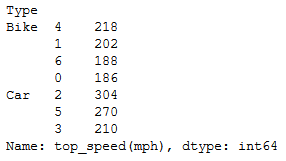Attention geek! Strengthen your foundations with the Python Programming Foundation Course and learn the basics.

To begin with, your interview preparations Enhance your Data Structures concepts with the Python DS Course.

My Personal Notes arrow_drop_upCheck out this Author's contributed articles.

If you like GeeksforGeeks and would like to contribute, you can also write an article using contribute.geeksforgeeks.org or mail your article to contribute@geeksforgeeks.org. See your article appearing on the GeeksforGeeks main page and help other Geeks.

Please Improve this article if you find anything incorrect by clicking on the "Improve Article" button below.

Article Tags :

Be the First to upvote.

Please write to us at contribute@geeksforgeeks.org to report any issue with the above content.